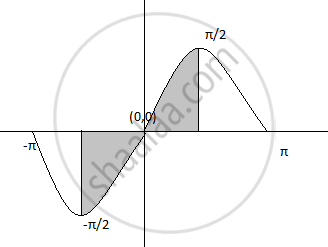# Find the area of the region bounded by the curve y = sinx, the lines x=-π/2 , x=π/2 and X-axis - Mathematics and Statistics

Sum

Find the area of the region bounded by the curve y = sinx, the lines x=-π/2 , x=π/2 and X-axis

#### Solution

Required area= where y=sinx , 0 ≤ x ≤ pi/2A = int_0^(pi/2) sin  x  dx

= [- "cos x"]_0^(pi/2)

= "cos" pi/2 + "cos"  0 = 0 + 1 = 1 sq.units

Concept: Area of the Region Bounded by a Curve and a Line
Is there an error in this question or solution?